# Assignments

1. Younger people cruises
The data for this assignment are listed in the Excel file Younger people cruises.
The cruise ship business is rapidly increasing. Although cruises have long been associated with seniors, it now appears that younger people are choosing a cruise as their vacations. To determine whether this is true, an executive for a cruise line sampled passengers 2 years ago and this year and determined their ages.
a. Formulate the null hypothesis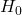and the alternative hypothesis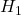.
b. For the inference about comparing the population means of two independent populations (which is the case here) it is important to know whether the population variances are equal. Explain why. A test determines that the population variances may be assumed to be equal. Which test is used and explain why it comes to this conclusion?
c. Do these data allow the executive to infer that cruise ships are attracting younger customers at a 1% significance level? Explain your answer.
d. Estimate with 99% confidence the difference in ages between this year and 2 years ago.

Solution

2. Reaction time cell phones
The data for this assignment are listed in the Excel file Reaction time cell phones.

Studies seem to indicate that using a cell phone while driving is dangerous. One reason for this is that the driver’s reaction time may slow while he or she is talking on the phone.
Researchers at Miami (Ohio) University measured the reaction times of a sample of drivers who owned a cell phone. A number of them (the Phone data) were tested while on the phone, and the others (the No Phone data) were tested while not on the phone.
We want to find out whether the reaction times are slower for drivers using cell phones. The significance level is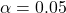.
a. Explain why we should consider the two groups of drivers as two independent populations.
b. An important decision is whether you may assume equal or unequal variances of both populations. It appears that it is allowed to assume equal variances. How could you find out that you are allowed to use the equal-variances test statistic?
c. What is the equal-variance test statistic and explain the formula.
d. Can we conclude that reaction times are slower for drivers using cell phones?

Solution.
a. Data from one group are independent from the data from the other group. The sizes of these two samples are not equal.
b. The two tail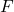-test is for testing equal variances with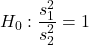and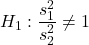. With Excel select Data, Data Analysis,-test Two-Sample for Variances. The test statistic is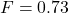with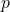-value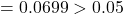, so there is no reason to reject, and equal variances may be assumed.
c.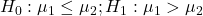The test statistic (pooled variance) is: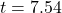and the-value(may be computed either manually or by Excel).
d. The value of the test statistic is far in the rejection region and thus we reject. We may conclude that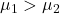. The reaction times are slower for drivers using cell phones.

3. Difference in debt
The data for this assignment are listed in the Excel file Difference in debt.
Are Americans more deeply in debt this year compared to last year? To help answer this question, a researcher randomly sampled the same Americans (heads of a household) this year and last year. For each, the ratio of debt payments to household income was recorded.
a. What kind of test is most appropriate?
b. Formulate the test statistic, and the null hypothesisand the alternative hypothesis.
c. Can we infer at a 5% significance level that the ratios are higher this year than last?

Solution.
a. In this case we are dealing with a matched pairs experiment: same heads of the household are sampled twice.
b. The test statistic of a matched pairs experiment is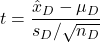, where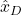denotes the sample mean of the differences,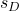the sample standard deviation and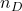the numbers of households. The null hypothesis is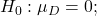.
c. The test statistic is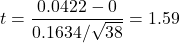. It is a one-sided test and thus the critical region is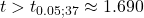. The test statistic is not in the rejection region and thus there is not enough evidence to conclude that the ratios are higher this year than last.
Also, Data Analysis | t-test matched pairs experiment can be used, with almost similar results.

4. Healthy companies
The data for this assignment is listed in the Excel file Healthy companies.
In what ways are companies that fail different from those that are healthy?
To answer this question, one study compared various characteristics of 68 healthy and 33 failed mutually independent firms. One of the variables was the ratio of current assets to current liabilities. Roughly speaking, this is the amount that the firm is worth divided by what it owes.
The question of interest is whether or not the mean ratio of current assets to current liabilities is different for the two groups. The significance level is 5%.
a. Formulate the null and alternative hypotheses.
b. The population variances of both populations may be assumed to be not equal. How can you verify this and give the relative results.
c. Based on the result of b. we may apply the ‘compare two means’ t-test. Explain how you did this and give the result.
Excel may be used whenever you like.

Solution.
a.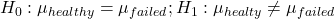.
b. Apply the-test Two-Sample for Variances. It appears that the variance of the healthy firms equals 0.4087 and of the failed firms 0.2314. so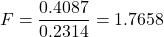. The critical value equals. Since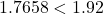the test statisticdoes not fall into the rejection region. There is evidence that the variances do not differ.
c. The test statistic is (according to Excel’s t-Test: Two-Sample Assuming Equal Variances): 7.17. The rejection region isThe test statistic falls into the rejection region andmust be rejected: the means are not equal.

5. Brass mailboxes
The data for this assignment are listed in the Excel file Brass mailboxes.
A manufacturer of outdoor brass mailboxes has received numerous complaints about premature corrosion. The manufacturer has identified the cause of the problem as the low-quality lacquer used to coat the brass. He decides to replace his current lacquer supplier with one of five possible alternatives. To judge which is the best, he uses each of the five lacquers to coat 25 brass mailboxes and puts all 125 mailboxes outside. He records, for each, the number of days until the first sign of corrosion is observed.
The manufacturer wants an answer to the question: is there sufficient evidence at the 1% significance level to allow him to conclude that differences exist between the five lacquers? In order to find an answer to this question he applies ANOVA.
Normality of the populations is assumed.
a. Use Descriptive Statistics in Data Analysis to determine the variances of the data of each lacquer. Take the two variances that differ most: V(lacquer 2) and V(lacquer 5) and show that these two variances may be assumed to be equal at the 5% significance level. Give the results.
b. We use Excel to apply ANOVA. Determine the null and alternative hypothesis and find the-value and the corresponding-value. Explain why the null hypothesis is rejected.

Solution.
a.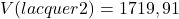and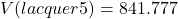.
The result of Excel’s two-sample variance test is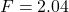with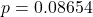. We may conclude at the 5% significance level that lacquer 2 and lacquer 5 have equal variances and thus that the variances of all lacquers may be assumed to be equal.
b.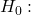all means are equal,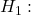at least one mean differs from the others. The ANOVA test has the result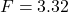with-value 0.0129 (>0.01). Since the manufacturer wants to use a 1% significance level there is not enough evidence to allow the manufacturer to conclude that differences exist between the five lacquers.

0